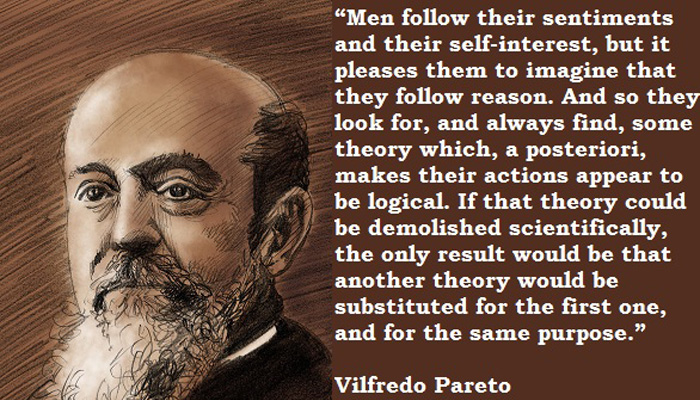## trimming poor importance samplers with Pareto scissorsLast week A while ago, Aki Vehtari and Andrew Gelman arXived a paper on self-normalised importance sampling estimators, Pareto smoothed importance sampling. That I commented almost immediately and then sat on, waiting for the next version. Since the two A’s are still working on that revision, I eventually decided to post the comments, before a series of posts on the same issue. Disclaimer: the above quote from and picture of Pareto are unrelated with the paper!

A major drawback with importance samplers is that they can produce infinite variance estimators. Aki and Andrew compare in this study the behaviour of truncated importance weights, following a paper of Ionides (2008) Andrew and I had proposed as a student project last year, project that did not conclude. The truncation is of order √S, where S is the number of simulation, rescaled by the average weight (which should better be the median weight in the event of infinite variance weights). While this truncation leads to finite variance, it also induces a possibly far from negligible bias, bias that the paper suggests to reduce via a Pareto modelling of the largest or extreme weights. Three possible conclusions come from the Pareto modelling and the estimation of the Pareto shape k. If k<½, there is no variance issue and truncation is not necessary; if ½<k<1, the estimator has a mean but no variance, and if k>1, it does not even has a mean. The latter case sounds counter-intuitive since the self-normalised importance sampling estimator is the ratio of an estimate of a finite integral by an estimate of a positive constant… Aki and Andrew further use the Pareto estimation to smooth out the largest weights as estimated quantiles. They also eliminate the largest weights when k comes close to 1 or higher values.

On a normal toy example, simulated with too small a variance, the method is seen to reduce the variability if not the bias. In connection with my above remark, k does never appear as significantly above 1 in this example. A second toy example uses a shifted t distribution as proposal. This setting should not induce a infinite variance problem since the inverse of a t density remains integrable under a normal distribution, but the variance grows with the bias in the t proposal and the Pareto index k as well, exceeding the boundary value 1 in the end. Similar behaviour is observed on a multidimensional example.

The issue I have with this approach is the same I set to Andrew last year, namely why would one want to use a poor importance sampler and run the risk of ending up with a worthless approximation? Detecting infinite variance estimation is obviously an essential first step step to produce reliable approximation but a second step would to seek a substitute for the proposal in an automated manner, possibly by increasing the tails of the original one, or in running a reparameterisation of the original problem with the same proposal. Towards thinner tails of the target. Automated sounds unrealistic, obviously, but so does trusting an infinite variance estimate. If worse comes to worse, we should acknowledge and signal that the current sampler cannot be trusted. As in statistical settings, we should be able to state we cannot produce a satisfactory solution (and hence need more data or different models).

This site uses Akismet to reduce spam. Learn how your comment data is processed.